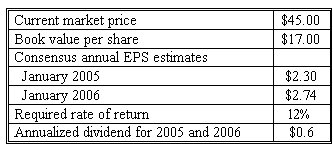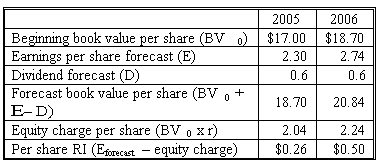### Seeing is believing!

Before you order, simply sign up for a free user account and in seconds you'll be experiencing the best in CFA exam preparation.

##### Subject 3. The Residual Income Valuation Model
The General Residual Income Model

An important assumption behind the residual income model is clean surplus relation which states the relationship among earnings, dividends and book value per share:

BV1 = BV0 + EPS1 - D1

This means all changes in book value can be explained by the company's accounting profits and distributions to shareholders. In later readings of this study session we will explore the adjustments that need to be made for the model to work, if this relationship does not hold.

If the clean surplus relationship holds, we can derive residual income model from dividend discount model:

V0 = D1 / (1 + r) + D2 /(1 + r)2 + ...

Since Dt+1 = Bt + EPSt+1 - Bt+1:

V0 = (B0 + EPS1 -B1) / (1 + r) + (B1 + EPS2 - B2) / (1 + r)2 + ...

We rewrite this equation to arrive to the residual income model:

V0 = B0 + Σ(RIt / (1 + r)t) = B0 + Σ ((Et - r x Bt-1) / (1 + r)t)

where

• V0 - value of a company's stock.
• B0 - current book value per share.
• Bt - forecasted book value per share at the end of period t.
• r - cost of equity.
• Et - expected EPS for period t.
• RIt - residual income per share for period t.

An alternative expression for this model would be:

V0 = B0 + Σ ((ROEt - r) x Bt-1/ (1 + r)t)

The RI model breaks intrinsic value of a stock into two components:

• The current book value of equity;
• The present value of expected future RI.

The idea is simple: forecast residual incomes for every future period, discount them at the required rate of return on equity, then add the current book value per share, and you'll get the value of the company's stock.

As we have noted earlier, residual income can be calculated as EPS decreased by equity charge r Bt-1. To forecast future residual earnings, we need to estimate EPS and equity charges for every future period. Equity charges can be determined if we have future book values per share and required rate of return on equity. Addition to book value each year equals the difference between EPS and dividends per share.

Example: Per share residual income forecasts.

The following data is for a firm as of January, 2005.What is the forecast residual income for fiscal years ended January 2005 and January 2006?Example: Using the RI model.

An analyst has determined that Tallulah Energy has a required rate of return of 11% and has compiled the following information about the firm for the next three years. After 2007, the firm will cease doing business and will distribute a liquidating dividend.Using the RI methodology, the value of a share is: V0 = \$6.5 + 0.58/1.111 + 0.71/1.112 + 1.27/1.113 = \$8.53.

\$8.53 is also the discounted value of the dividends. In theory, RI is consistent with DCF. In most cases, however, value is recognized earlier under the RI approach. A large part of the stock's intrinsic value is recognized immediately - it's the current book value per share. This is a practical advantage since residual income valuations are thus less sensitive to remote future terminal value estimates. The shorter forecast horizon warrants more precise valuation.